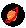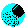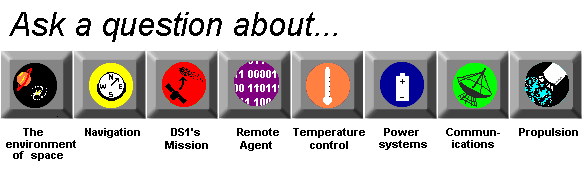# Space Environment

## How do scientists know what the path of an object in space will be?

Scientists know the basic laws of physics or movement rules that objects in space follow. Because of this, if a scientist knows how big something is (how much mass an object has) how fast its going, the direction its going and what it will be going near, that scientist can figure out what its flight will look like.

A very basic principle of physics says that if something is going in a straight line it has something called inertia that will keep it going in a straight line at the same speed unless a force makes it change or stop. The bigger (more massive) something is, the more inertia it has and the harder it is to stop it or change its course. That's why if you drop a bowling ball on a glass table, it will keep going through the table, while a marble will be stopped by it.

Gravity is the most important force affecting movement in space. The strength of gravity's pull between two objects depend on how big they are and how close they are. (Generally one object is a lot bigger than the other, and so most of the gravitational pull comes from one to the other.)

If a scientist knows how big something is, how fast it is moving, and how close it is to other things with gravity it is, the scientist can get out a calculator and a math book to see how gravity and inertia will act on the object. These two factors (gravity and inertia) will usually completely determine the path of the object.What is an orbit?Is there gravity in space?What is gravity?How does propulsion work?What is mass?What is inertia?What is resistance?Why do mass and distance affect gravity?